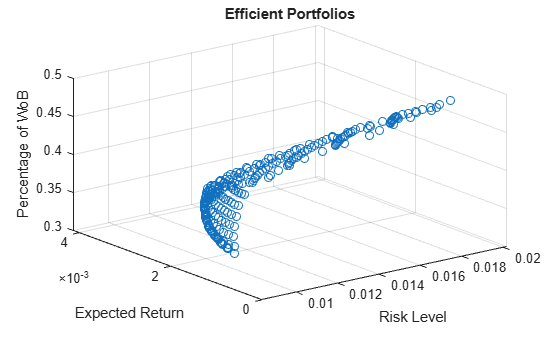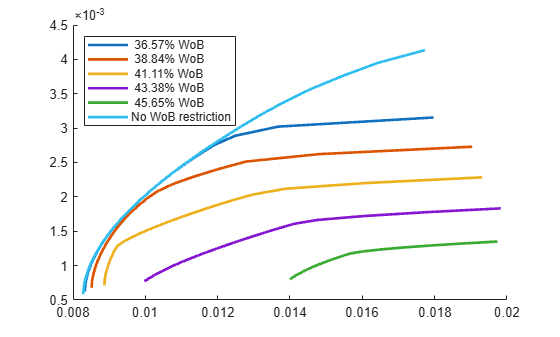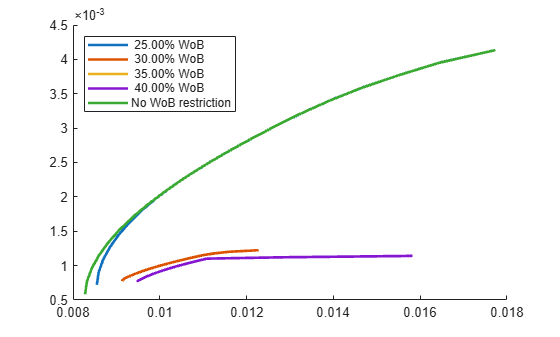# Portfolio Optimization Using Social Performance Measure

Use a `Portfolio` object to minimize the variance, maximize return, and maximize the average percentage of women on a company's board. The same workflow can be applied with other Environmental, Social and Governance (ESG) criteria, such as an ESG score, a climate, or a temperature score.

The goal of this example is to find portfolios that are effcient in the sense that they minimize the variance, maximize return, and maximize the average percentage of women on the board of directors. To find the average percentage of women on a company's board (`WoB`) for a given portfolio, this example uses a weighted sum of the percentages of `WoB` for each individual asset, where the weights are given by the amount invested in each asset for the portfolio. By defining the average percentage of `WoB` this way, the `WoB` function is linear with respect to the weights.

```load CAPMuniverse % Assume that the percentage of women on the board of directors per % company are as follows WoB = [0.2857; 0.5; 0.3; 0.2857; 0.3077; 0.2727; ... 0.4167; 0.2143; 0.3; 0.4167; 0.3077]; table(WoB,'VariableNames',{'WoB'},'RowNames',Assets(1:11))```
```ans=11×1 table WoB ______ AAPL 0.2857 AMZN 0.5 CSCO 0.3 DELL 0.2857 EBAY 0.3077 GOOG 0.2727 HPQ 0.4167 IBM 0.2143 INTC 0.3 MSFT 0.4167 ORCL 0.3077 ```

### Create `Portfolio` Object

Create a standard `Portfolio` object and incorporate the list of assets and estimate the moments of the assets' returns from the data. Use `setDefaultConstraints` to set the default mean-variance portfolio constraints. These constraints require fully invested, long-only portfolios where the nonnegative weights must sum to `1`.

```% Create portfolio with default constraints p = Portfolio('AssetList',Assets(1:11)); p = estimateAssetMoments(p, Data(:,1:11)); p = setDefaultConstraints(p);```

### Set Group Constraints

Use `getGroups` to include group contraints. The first group constraint ensures that the weights invested in mixed retail (Amazon and eBay) are at least 15%. The second group constraint ensures that the weights invested in computer companies (Apple, Dell and HP) are between 25% and 50%.

```% Group constraints G = [0 1 0 0 1 0 0 0 0 0 0; 1 0 0 1 0 0 1 0 0 0 0]; LowG = [0.15; 0.25]; UpG = [Inf; 0.5]; p = setGroups(p, G, LowG, UpG);```

Find the minimum and maximum percentage of `WoB` that a portfolio can attain given these extra group constraints. This is done using the `estimateCustomObjectivePortfolio` function with the average percentage of women on a company's board as the objective function.

```% Set average WoB as the objective objFun = @(x) WoB'*x;```

Find the portfolio with the minimum average percentage of `WoB` with the group constraints using `estimateCustomObjectivePortfolio` with the function handle `objFun`.

```% Minimum percetage of women on the board wgt_minWoB = estimateCustomObjectivePortfolio(p,objFun); minWoB = objFun(wgt_minWoB)```
```minWoB = 0.2462 ```

Find the portfolio with the maximum average percentage of WoB with the group constraints using `estimateCustomObjectivePortfolio` with the name-value argument `ObjectiveSense` set to `maximize`.

```% Maximum percentage of women on the board wgt_maxWoB = estimateCustomObjectivePortfolio(p,objFun,... ObjectiveSense="maximize"); maxWoB = objFun(wgt_maxWoB)```
```maxWoB = 0.4792 ```

### Compute and Plot the Efficient Surface

Define a grid of `WoB` percentages such that `minWoB` $=$ `targetWoB(1)` $\le \dots \le$ `targetWoB(N)` $=$ `maxWoB`.

```N = 20; % Size of grid targetWoB = linspace(minWoB,maxWoB,N);```

Use `setInequality` to set the percentage of `WoB` as a constraint. The coefficients of the linear constraint should be the `WoB` percentages associated to each asset, and the right-hand side should be the target portfolio `WoB`. The convention of the inequality is $\le$. Since the goal is to maximize portfolio WoB, then the target WoB should be a lower bound for the portfolio WoB. Therefore, the signs of the coefficients and the right-hand side of the added inequality should be flipped.

```Ain = -WoB'; bin = -minWoB; % Start with the smallest WoB p = setInequality(p,Ain,bin);```

For each target `WoB`, `targetWoB(i)`, find the efficient mean-variance frontier using `estimateFrontier`. At each iteration, the right-hand side of the `WoB` portfolio constraint should be changed to ensure that the returned portfolios achieve at least the target `WoB`. This method returns the weights of the portfolios on the mean-variance efficient frontier that have a `WoB` of at least `targetWoB(i)`. Using the weights obtained for each target `WoB`, compute the portfolios' expected return, risk, and percentage of `WoB`.

```% Get efficient surface values prsk = cell(N,1); pret = cell(N,1); pWoB = cell(N,1); for i = 1:N p.bInequality = -targetWoB(i); pwgt = estimateFrontier(p,N); [prsk{i},pret{i}] = estimatePortMoments(p,pwgt); pWoB{i} = pwgt'*WoB; end```

Plot the efficient portfolios.

```% Plot efficient surface scatter3(cell2mat(prsk),cell2mat(pret),cell2mat(pWoB)) title('Efficient Portfolios') xlabel('Risk Level') ylabel('Expected Return') zlabel('Percentage of WoB')```To visualize the tradeoff between a portfolio's average percentage of `WoB` and the traditional mean-variance efficient frontier, a set of contour plots are computed for some target `WoB` percentages using the `plotContours` function in Local Functions.

```nC = 5; % Number of contour plots minContour = max(pWoB{1}); % WoB values lower than this return % overlapped contours. % Plot contours plotContours(p,minContour,maxWoB,nC,N)```### Exclusion Examples

Instead of requiring a specific level for the portfolio's average percentage of `WoB`, the goal is to find the traditional mean-variance efficient frontiers while excluding assets that have a percentage of WoB lower than a given threshold. You can plot the exclusion using the `plotExclusionExample` function in Local Functions.

```% Remove the average percetage of WoB constraint p.AInequality = []; p.bInequality = []; % Set of thresholds for excluding assets thresholdWoB = 0.25:0.05:0.40; % Plot exclusion example plotExclusionExample(p,WoB,thresholdWoB,N)```The differences between this approach and the one presented in the previous section are quite evident. Requiring all the assets to have a `WoB` percentage of at least 35% gives an efficient frontier that can achieve a return of at most around $1.2{\left(10\right)}^{-3}$. On the other hand, requiring only that the portfolio's average percentage of `WoB` is 36.57% gives the possibility to reach a return of around $3.2{\left(10\right)}^{-3}$, almost 2.5 times the return obtained when excluding assets. To better show the differences between these two approaches, compute the maximum return achieved for a given standard deviation for the two ways of including the percentage of `WoB` requirements to the portfolio.

#### Approach 1

In the first approach, exclude all assets with a `WoB` percentage lower than 33% and find the portfolio of maximum return that has a standard deviation of at most `0.012`.

```% Select assets to exclude ub = zeros(p.NumAssets,1); ub(WoB >= 0.33) = 1; p.UpperBound = ub; % Estimate the return for a risk level of 0.012 pwgt_exclude = estimateFrontierByRisk(p,0.012); ret_exclude = estimatePortReturn(p,pwgt_exclude)```
```ret_exclude = 0.0011 ```
```% Return constraints to the original portfolio p.UpperBound = [];```

#### Approach 2

For the second approach, ensure that the average `WoB` percentage is of at least 33% and find the portfolio of maximum return that has a standard deviation of at most `0.012`.

```% Include WoB constraint into the portfolio p = addInequality(p,-WoB',-0.33); % Estimate the return for a risk level of 0.012 pwgt_avgWoB = estimateFrontierByRisk(p,0.012); ret_avgWoB = estimatePortReturn(p,pwgt_avgWoB)```
```ret_avgWoB = 0.0028 ```
```% Return constraints to the original portfolio p.AInequality = []; p.bInequality = [];```

Compute the increase in return between these two approaches.

`ret_increase = (ret_avgWoB-ret_exclude)/ret_exclude`
```ret_increase = 1.5202 ```

This `ret_increase` value shows that the return from the approach that only bounds the portfolio's average WoB percentage instead of excluding certain assets has a return 152% higher (for the same risk level). Hence, when tackling problems with more than two objectives, excluding assets that do not meet a certain criteria might not be the best option. Instead, a weighted sum of the criteria of interest might show better results.

### Local Functions

```function [] = plotContours(p,minWoB,maxWoB,nContour,nPort) % Set of WoB levels for contour plot contourWoB = linspace(minWoB,maxWoB,nContour+1); % Compute and plot efficient frontier for each value in contourWoB figure; hold on labels = strings(nContour+1,1); for i = 1:nContour p.bInequality = -contourWoB(i); plotFrontier(p,nPort); labels(i) = sprintf("%6.2f%% WoB",contourWoB(i)*100); end % Plot the "original" mean-variance frontier, i.e., the frontier % without WoB requierements p.AInequality = []; p.bInequality = []; plotFrontier(p,nPort); labels(i+1) = "No WoB restriction"; legend(labels,'Location','northwest') hold off end function [] = plotExclusionExample(p,WoB,thresholdWoB, ... nPort) % Compute and plot efficient frontier excluding assets that are below % the WoB threshold nT = length(thresholdWoB); figure; hold on labels = strings(nT+1,1); for i=1:nT ub = zeros(p.NumAssets,1); % Only select assets above WoB threshold ub(WoB >= thresholdWoB(i)) = 1; p.UpperBound = ub; plotFrontier(p,nPort); labels(i) = sprintf("%6.2f%% WoB",thresholdWoB(i)*100); end % Plot the "original" mean-variance frontier, i.e., the frontier % without the WoB threshold p.UpperBound = []; plotFrontier(p,nPort); labels(i+1) = "No WoB restriction"; legend(labels,'Location','northwest') hold off end ```